Working with Fractions

Things to keep in mind when using your graphing calculator with fractions:

 1. Yikes!  There is NO fraction key!! Classic Mode: Don't panic!  Just remember that the fraction bar means "divide". Enter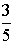as  " 3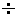5 ".              The calculator will display .6

MathPrint Mode:
The MathPrint Mode has templates to help you enter fractions quickly. Press ALPHA and F1. Make a choice and use arrow keys to navigate.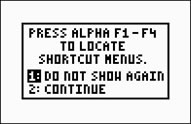Choosing #1 will place a template on the screen for entering a fraction.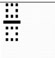Choosing #2 will place a template on the screen for entering a mixed number.Choosing #3 will convert an entry or a previous answer to a mixed number or an improper fraction.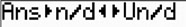2. Convert Decimals to Fractions. If you need to convert your decimal answer to a fraction, choose the MATH key and #1►Frac.   The screen will show Ans►Frac.  Hit Enter.   (Note:  The fraction answer will always appear in fraction or improper form.  It will not be converted to a mixed number.) Classic Mode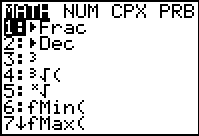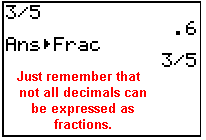MathPrint Mode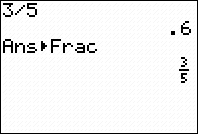In MathPrint, you may also enter fractions as shown in #1. Choosing #4 from the F1 menu, you can also change between fractions and decimals.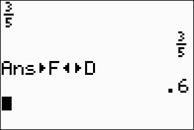3.
Mixed numbers may be entered directly.

 Classic Mode: A mixed number can be entered as it is read ---        " three AND (+) one half "Enter 3 + 1 / 2

Check out the two problems below.  Look carefully at the subtraction problem.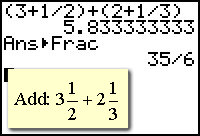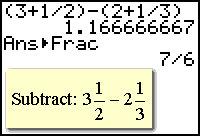Beware!! In the examples on the left, the use of parentheses was not needed when adding, but WAS needed when subtracting. If the parentheses was not used in the subtraction problem, only the 2 would have been subtracted (by order of operations) and the wrong answer would have resulted.

 MathPrint Mode: Mixed numbers can be entered directly using the F1 templates.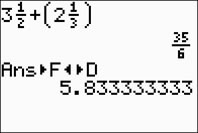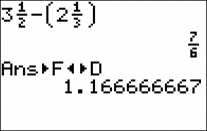The parentheses are entered automatically by the calculator AFTER you have entered the mixed numbers. Notice that it leaves the answers in fraction form.

 4. Mixed numbers entered as improper fractions. Classic Mode: If you have a tendency to "forget" your parentheses when dealing with mixed numbers, you should consider changing all mixed numbers to improper fractions.  This will avoid the need to worry about the parentheses.could also be ....could also be ....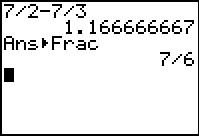5. Entering Algebraic Fractions Be careful when entering algebraic fractions. Consider this problem:Classic Mode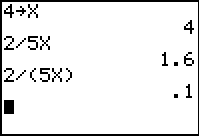For testing purposes, the value 4 was stored in x using the STO► key. Notice the different answers!!!  The calculator only recognizes division between the character immediately before and after the division symbol. Parentheses were needed for this problem (the second answer (.1) is correct.) MathPrint Mode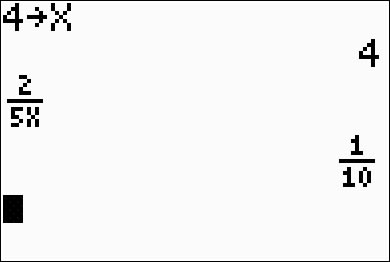The same problem as seen above will occur with MathPrint if you enter 2/5x. To avoid such problems, use the F1 template to enter the fraction. When using the template, the denominator will stay in place and avoid the problem.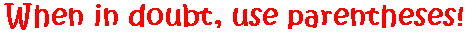Finding Your Way Around TABLE of  CONTENTS